# Swift Algorithm Club: Graphs with Adjacency List

Learn how to implement directed and undirected graphs in Swift using adjacency lists.

Every month, Kelvin Lau, Chris Pilcher and I feature a data structure or algorithm on this site. If you want to learn more about algorithms and data structures, follow along with us!

In this tutorial, you’ll learn the basics of graph theory, then create an adjacency list in Swift 3.

Note: New to the Swift Algorithm Club? Check out our getting started post first.

## Getting Started

A Graph is a useful data structure to capture relationships between objects, made up of a set of vertices paired with a set of edges.

In the graph below, the vertices represents the circles, and the edges are the lines between them. A vertex connects to other vertices by edges.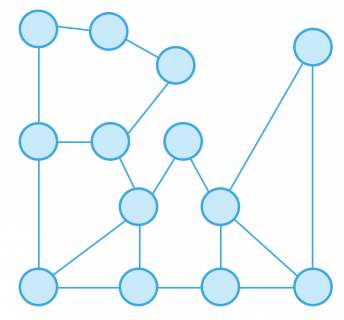Graphs can come in a variety of shapes and sizes.

### Weighted Graph

Take an airline for example. Imagine a network that shows varying routes for flights. Let the vertices represent the cities and the edges represent a possible route from city to city. Now you can associate a weight to every edge. From this network you can decipher the cheapest flights from San Francisco to Singapore.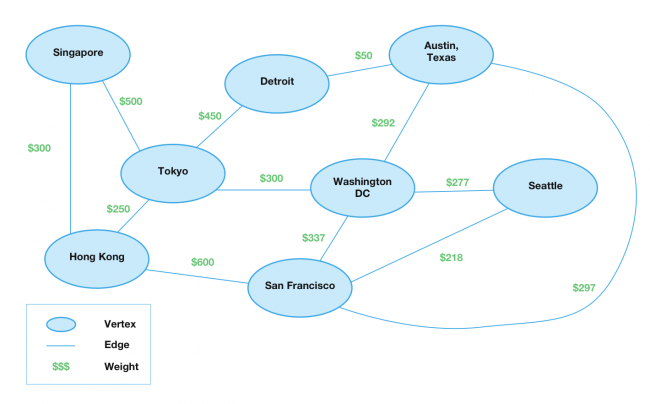The graph above represents a weighted graph where each edge has a numerical value.

### Directed and Undirected Graphs

A graph could also have direction. The first graph is a directed graph, where edges have direction. Imagine a flight from Toyko to Detroit, but no flight from Tokyo to Washington D.C.

A directed graph can also be bidirectional, where two vertices have two edges going back and forth. For example a flight from Singapore to Hong Kong, has a flight back as well.

The latter graph is an undirected graph, where there is no direction. In a way an undirected graph is just a directed graph that is bidirectional.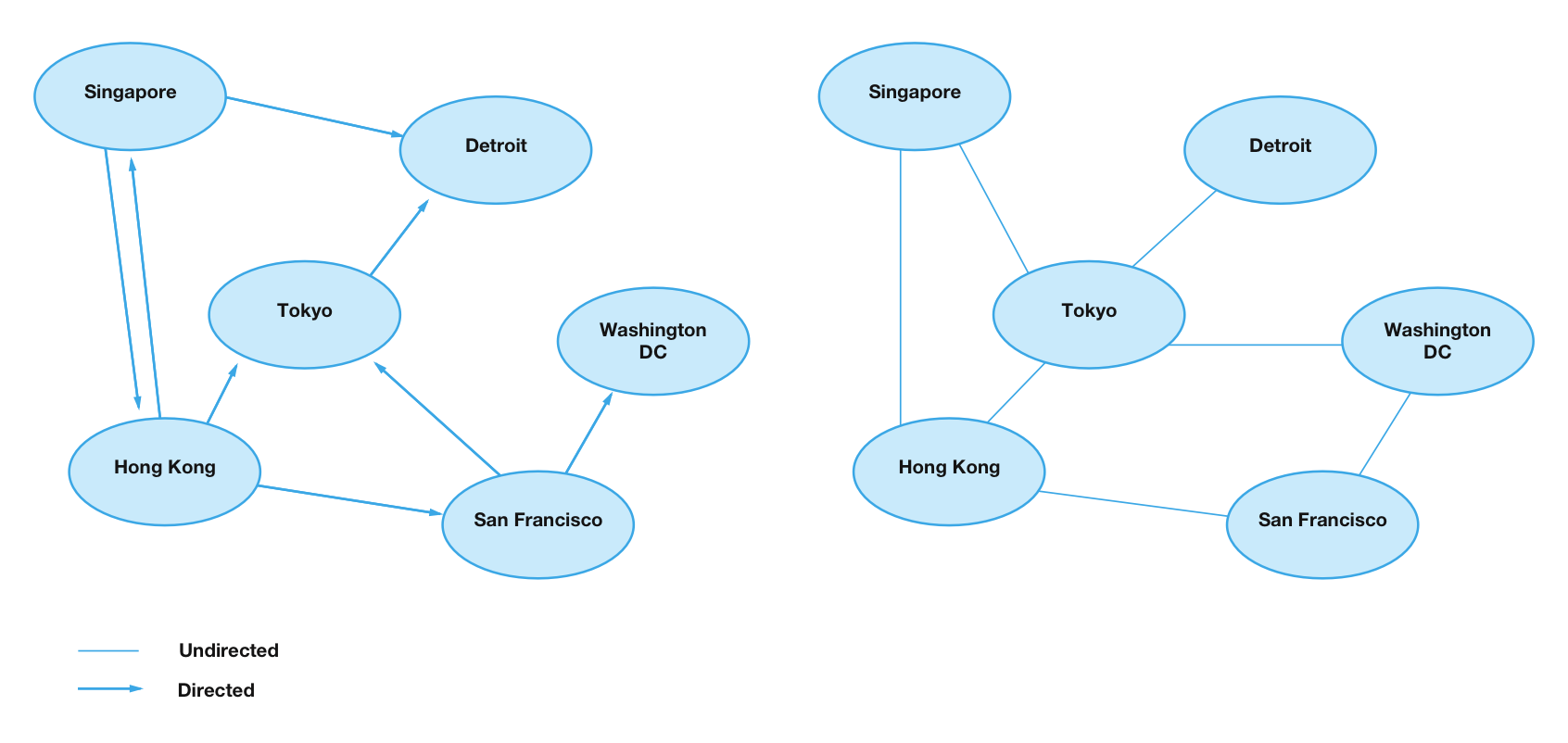You may have come across trees or linked-list data structures. They are a more simplified version of graphs.

## Representing a Graph

The two common ways to represent a graph is through an adjacency matrix or adjacency list. To get started with graphs, you will learn to create an adjacency list.

The basic idea of an adjacency list is you store every single vertex. Each vertex will hold an adjacency list. This describes the outgoing edges.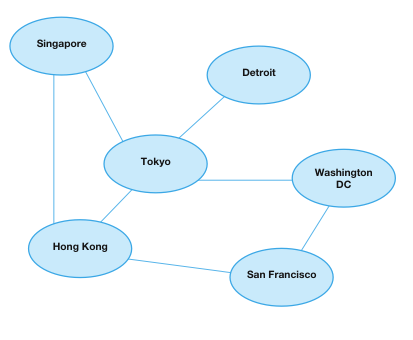The adjacency list below describes the flight network graph above.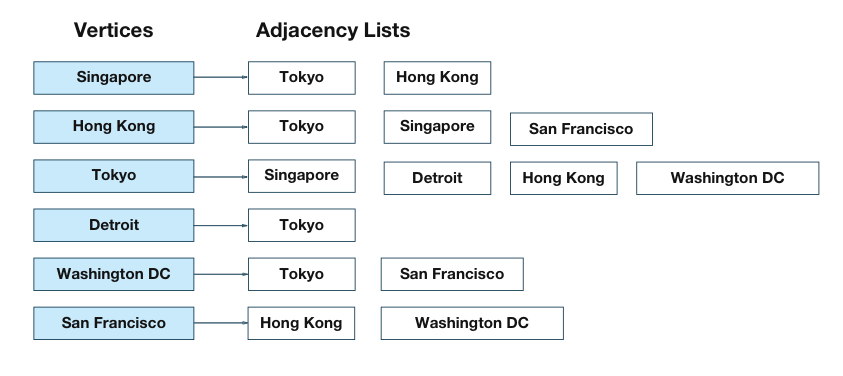As you can see the Singapore vertex has two edges associated with it. There are outgoing flights from Singapore to Toyko or Hong Kong.

### Approach

You can develop the adjacency list in many different ways. A few popular approaches are:

• Storing an array of arrays. The outer array represents vertices, providing an index. The inner array contains edges.
• Storing an array of linked-lists. With this approach, each index in the array represents a vertex. Each value in the array stores a linked-list. This is ideal if you need fast insertion or deletion times.
• Storing a dictionary of arrays. This is the approach you’ll take in this tutorial. Each key in the dictionary is a vertex, and each value is the corresponding array of edges.

Start by creating a new Swift playground called Graphs. In the Project Navigator (View\Navigators\Show Project Navigator, or ⌘-1), create a new file called Vertex.swift under the Sources group.

### Vertices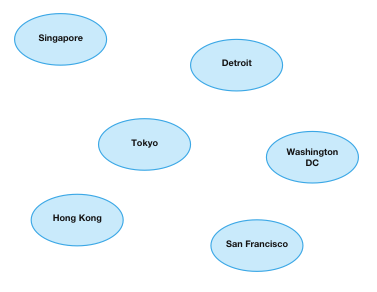The first thing that graphs need is a vertex. Add the following struct declaration in Vertex.swift:

```public struct Vertex<T: Hashable> {
var data: T
}
```

You’ve declared a struct named `Vertex`. The vertex holds a generic type called `data`. So now the vertex can represent pretty much any relationship, whether it’s airline flights, a person, or street addresses.

Next since you are storing a vertex as a custom key in a dictionary, it has to conform to the `Hashable` protocol. Add the following code below:

```extension Vertex: Hashable {
public var hashValue: Int { // 1
return "\(data)".hashValue
}

static public func ==(lhs: Vertex, rhs: Vertex) -> Bool { // 2
return lhs.data == rhs.data
}
}
```

Going over the code above:

1. For `Hashable` conformance you must provide a `hashValue` property.
2. `Hashable` inherits from the `Equatable` protocol. You must also add an equal-to operator for the custom type.

Now add the following code within the same file:

```extension Vertex: CustomStringConvertible {
public var description: String {
return "\(data)"
}
}
```

The `CustomStringConvertible` protocol allows you to define a custom output. You will use this to verify your adjacency list later.

### Edges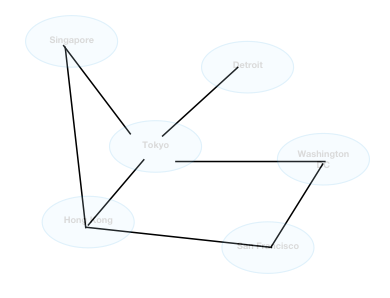Now to connect every vertex to another vertex, there needs to be an edge between them! Create a new file called Edge.swift under Sources group.

First add the following enum to Edge.swift

```public enum EdgeType {
case directed, undirected
}
```

The goal of the enum is to describe whether an edge between two vertices is a `directed` path, or `undirected` path.

Now add the following code after:

```public struct Edge<T: Hashable> {
public var source: Vertex<T> // 1
public var destination: Vertex<T>
public let weight: Double? // 2
}
```

Going over the properties in `Edge`:

1. There are many ways to represent an edge depending on your implementation. For this edge, it’s property includes two vertices, `source` and `destination`. The reason is because graphs can be directional. Two vertices are bidirectional, would need two edges between the pair of vertices.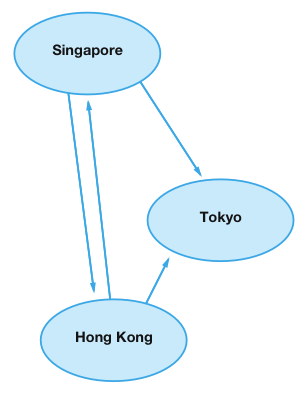Take this graph as an example. The flight between Singapore and Hong Kong has two edges between the pair of flights. This means the flight goes both ways. But, there is only one edge between Singapore and Tokyo. There are only flights that go from Singapore to Tokyo but not back.

2. An edge could also have a numerical weight. This is useful for future algorithms. You may want to find out how much it costs to fly from Hong Kong to Tokyo.

Like in the `Vertex` struct, add the following code in Edge.swift:

```extension Edge: Hashable {

public var hashValue: Int {
return "\(source)\(destination)\(weight)".hashValue
}

static public func ==(lhs: Edge<T>, rhs: Edge<T>) -> Bool {
return lhs.source == rhs.source &&
lhs.destination == rhs.destination &&
lhs.weight == rhs.weight
}
}
```

`Edge` conforms to the `hashable` protocol. For similar reasons to the `Vertex` struct.

### Graphable Protocol

Now that you have defined your `vertex` and `edge`. You have one more thing you have to do, which is to create an interface to build a graph.

To do this, create a new file called Graphable.swift under Sources group and add the following code:

```protocol Graphable {
associatedtype Element: Hashable // 1
var description: CustomStringConvertible { get } // 2

func createVertex(data: Element) -> Vertex<Element> // 3
func add(_ type: EdgeType, from source: Vertex<Element>, to destination: Vertex<Element>, weight: Double?) // 4
func weight(from source: Vertex<Element>, to destination: Vertex<Element>) -> Double? // 5
func edges(from source: Vertex<Element>) -> [Edge<Element>]? // 6
}
```

Going over the protocol:

1. The protocol requires an `associatedType` called `Element`. This allows the protocol to be generic, to hold any type.
2. The `description` property lets you define custom behavior to print out the contents of a graph. This can help with debugging!
3. `createVertex(data:)` provides a utility method to create a vertex.
4. `add(_:from:to:weight:)` provides a utility to add an edge between two vertices. You can specify the weight as well as whether an edge is directed or undirected.
5. `weight(from:to:)` provides a utility to get the weight of an edge between two vertices.
6. `edges(from:)` provides a utility to retrieve all the edges that `source` vertex connects to.

On to implementing the adjacency list!

First create a new file called AdjacencyList.swift within the Sources group.

```open class AdjacencyList<T: Hashable> {
public var adjacencyDict : [Vertex<T>: [Edge<T>]] = [:]
public init() {}
}
```

You will use `adjacencyDict` to store your graph. `adjacencyDict` is a dictionary. The key is a `Vertex` and the value is an array of edges.

Next add the following after the class declaration:

```extension AdjacencyList: Graphable {
public typealias Element = T
}
```

Since the `AdjacencyList` is also generic, the `associatedType` is set to `T`

Note: You may get some errors, because you have yet to conform to the `Graphable` protocol.

### Creating Vertices

To create a vertex add the following code within the `extension` right after the `Element` generic property:

```public func createVertex(data: Element) -> Vertex<Element> {
let vertex = Vertex(data: data)

}

return vertex
}
```

Based on the data passed in you create a `Vertex`. You check to see if the vertex already exists. If it doesn’t you initialize an array of edges and return the vertex.

### Creating Edges

Recall that there are graphs that are directed and graphs that are undirected.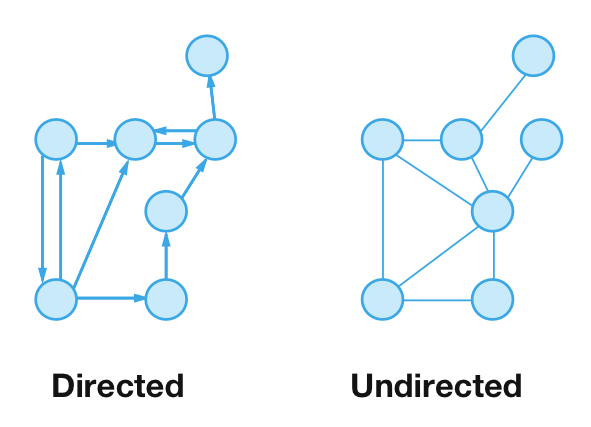First add the following helper method after `init()`:

```fileprivate func addDirectedEdge(from source: Vertex<Element>, to destination: Vertex<Element>, weight: Double?) {
let edge = Edge(source: source, destination: destination, weight: weight) // 1
}
```

This method takes two vertices, a source and destination with a weight. Let’s go over the code:

1. Creates an edge.
2. Retrieves the array of edges affiliated with source vertex and append the edge to the array.

Now add the following method right after `addDirectedEdge(from:to:weight:)`:

```fileprivate func addUndirectedEdge(vertices: (Vertex<Element>, Vertex<Element>), weight: Double?) {
let (source, destination) = vertices
addDirectedEdge(from: source, to: destination, weight: weight)
addDirectedEdge(from: destination, to: source, weight: weight)
}
```

In an undirected graph, you can treat it as a directed graph that goes both ways. You call
`addDirectedEdge(from:to:weight:)` twice, but with the source and destination vertices swapped.

Now you can make use of the two helper methods! Add the following code in the `AdjacencyList` extension, right after `createVertex(data:)`:

```public func add(_ type: EdgeType, from source: Vertex<Element>, to destination: Vertex<Element>, weight: Double?) {
switch type {
case .directed:
addDirectedEdge(from: source, to: destination, weight: weight)
case .undirected:
}
}
```

`add(_:from:to:weight:)` checks to see if the type is `directed` or `undirected` and creates the correct edge.

### Retrieving information

You will now finish up the conformance to `Graphable` by providing ways to retrieve the weight.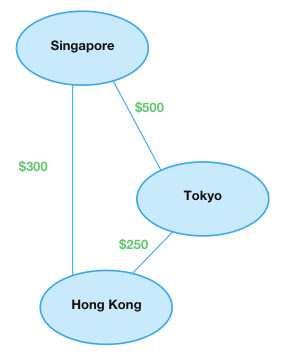How much is the flight from Singapore to Hong Kong?

Add the following code below the previous method:

```public func weight(from source: Vertex<Element>, to destination: Vertex<Element>) -> Double? {
guard let edges = adjacencyDict[source] else { // 1
return nil
}

for edge in edges { // 2
if edge.destination == destination { // 3
return edge.weight
}
}

return nil // 4
}
```

Let’s go over how to get the weight:

1. Retrieve all the edges from the `source` vertex. If no edges, return `nil`.
2. Loop through each edge.
3. Check to see if there is an edge that leads to the `destination` vertex.
4. Return nil if no weight found.

Next add the following code below:

```public func edges(from source: Vertex<Element>) -> [Edge<Element>]? {
}
```

`edges(from:)` is straightforward. You access the dictionary based on a given vertex and return the array of edges.

The final piece to the puzzle is visualizing the adjacency list. You must create the final method to fulfill the `Graphable` protocol.

```public var description: CustomStringConvertible {
var result = ""
for (vertex, edges) in adjacencyDict {
var edgeString = ""
for (index, edge) in edges.enumerated() {
if index != edges.count - 1 {
edgeString.append("\(edge.destination), ")
} else {
edgeString.append("\(edge.destination)")
}
}
result.append("\(vertex) ---> [ \(edgeString) ] \n ")
}
return result
}
```

The `description` property goes through every key-value pair in the dictionary. It prints out the vertex, and all the vertices it’s connected to by an edge.

The adjacency list is finally complete! You must be all excited to use your first graphing data structure!

### Testing out the Adjacency List

Now it’s time to go back to the flights pricing network example.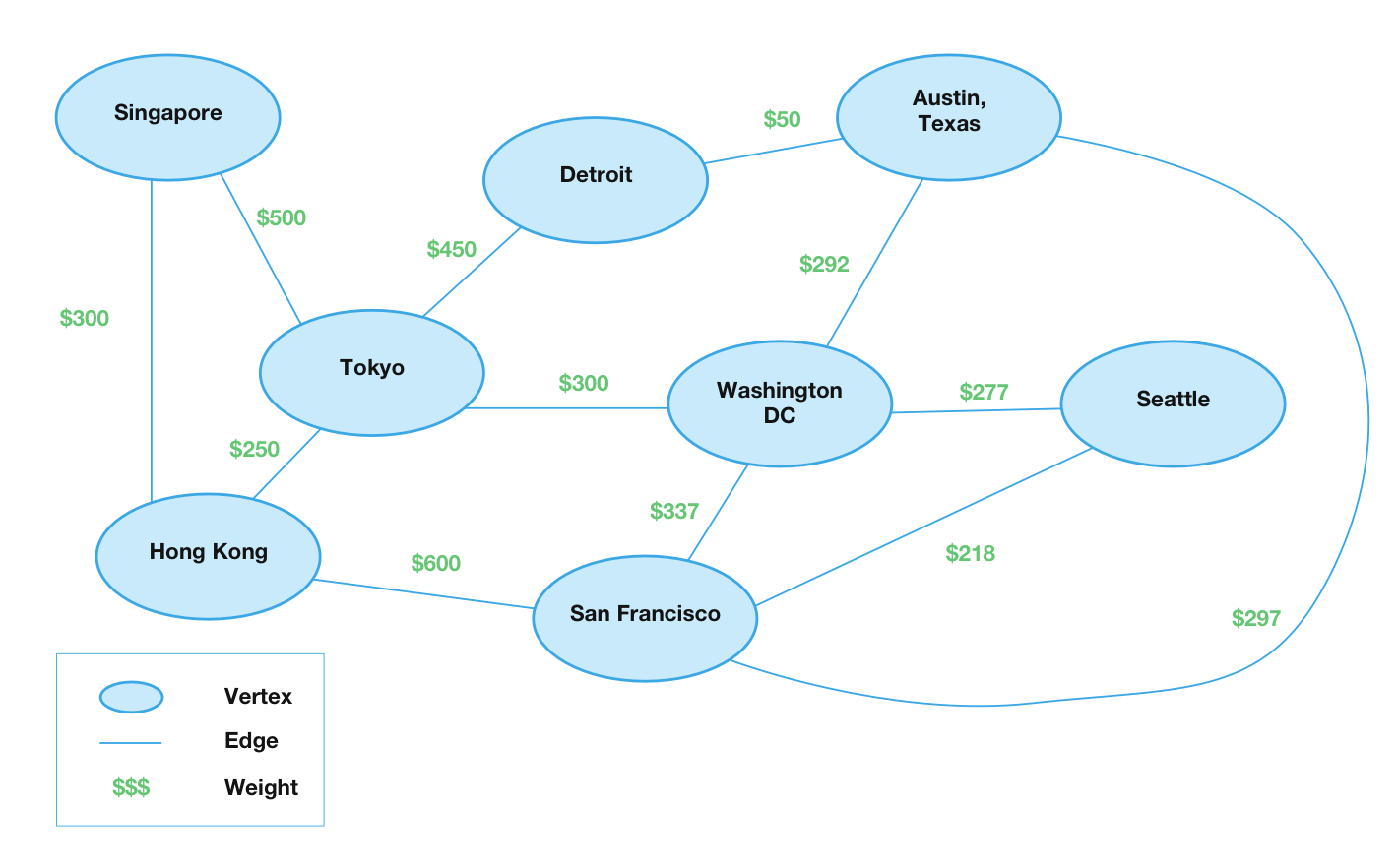Within the root Playground, add the following code:

```import UIKit
import XCPlayground

let hongKong = adjacencyList.createVertex(data: "Hong Kong")
let sanFrancisco = adjacencyList.createVertex(data: "San Francisco")
let washingtonDC = adjacencyList.createVertex(data: "Washington DC")
let austinTexas = adjacencyList.createVertex(data: "Austin Texas")

```

The code above creates the flight network pricing graph using the adjacency list. Swift Playgrounds should show the following output: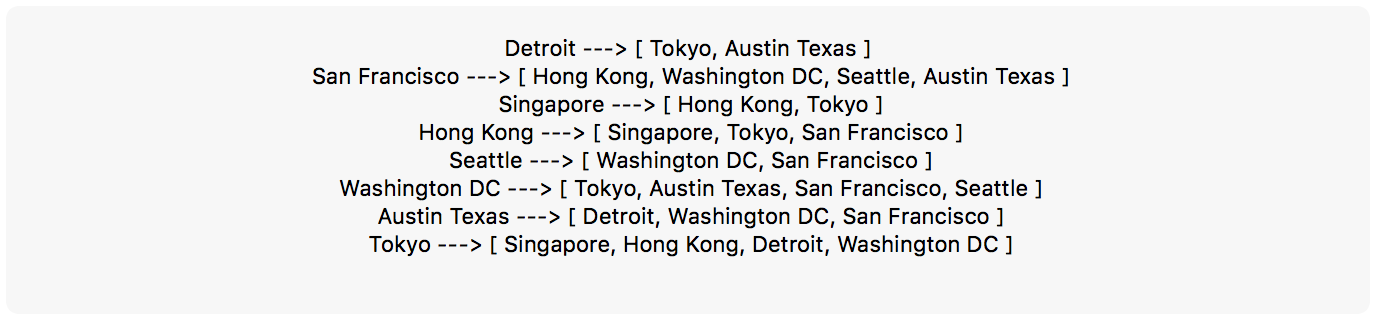Isn’t this cool? This is a visual description of an adjacency list! It shows you all the flights available from Austin Texas, all the flights from Toyko, and etc!

## Quiz Time

Use the graph created above to figure out how to use the adjacency list to solve the following problems:

How much does a ticket cost from Singapore to Toyko?

[spoiler title=”Solution”]

```adjacencyList.weight(from: singapore, to: tokyo)
```

[/spoiler]

What are the flights going out of San Francisco?
[spoiler title=”Solution”]

```if let flightsFromSanFrancisco = adjacencyList.edges(from: sanFrancisco) {
print("San Francisco Out Going Flights:")
print("--------------------------------")
for edge in flightsFromSanFrancisco {
print("from: \(edge.source) to: \(edge.destination)")
}
}
```

Flights going out are the following:

```San Francisco Out Going Flights:
--------------------------------
from: San Francisco to: Hong Kong
from: San Francisco to: Washington DC
from: San Francisco to: Seattle
from: San Francisco to: Austin Texas

```

[/spoiler]

## Where to Go From Here?

Here is the Swift Playground with all the code above. You can find alternative implementations and more information on graphs in the Swift Algorithm Club’s repository.

I hope you enjoyed this tutorial on making your first graph with an adjacency list! You have only scratched the surface of what graphs are capable of.

This was just one of the many algorithms from the Swift Algorithm Club’s repository. If you’re interested in more, check out the repo.

It’s in your best interest to know about algorithms and data structures. They’re solutions to many real world problems, and could come up during interviews. Plus it’s fun!

So stay tuned for many more tutorials from the Swift Algorithm club in the future. If you have any questions on graphs in Swift, please join the discussion below.

Note: The Swift Algorithm Club is always looking for more contributors. If you’ve got an interesting data structure, algorithm, or even an interview question to share, don’t hesitate to contribute! To learn more about the contribution process, check out our Join the Swift Algorithm Club article.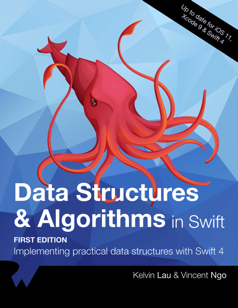If you enjoyed what you learned in this tutorial, why not check out our Data Structures and Algorithms in Swift book, available on our store?

In Data Structures and Algorithms in Swift, you’ll learn how to implement the most popular and useful data structures and when and why you should use one particular datastructure or algorithm over another. This set of basic data structures and algorithms will serve as an excellent foundation for building more complex and special-purpose constructs.

As well, the high-level expressiveness of Swift makes it an ideal choice for learning these core concepts without sacrificing performance.

• You’ll start with the fundamental structures of linked lists, queues and stacks, and see how to implement them in a highly Swift-like way.
• Move on to working with various types of trees, including general purpose trees, binary trees, AVL trees, binary search trees and tries.
• Go beyond bubble and insertion sort with better-performing algorithms, including mergesort, radix sort, heap sort and quicksort.
• Learn how to construct directed, non-directed and weighted graphs to represent many real-world models, and traverse graphs and trees efficiently with breadth-first, depth-first, Dijkstra’s and Prim’s algorithms to solve problems such as finding the shortest path or lowest cost in a network.
• And much, much more!

By the end of this book, you’ll have hands-on experience solving common issues with data structures and algorithms — and you’ll be well on your way to developing your own efficient and useful implementations.